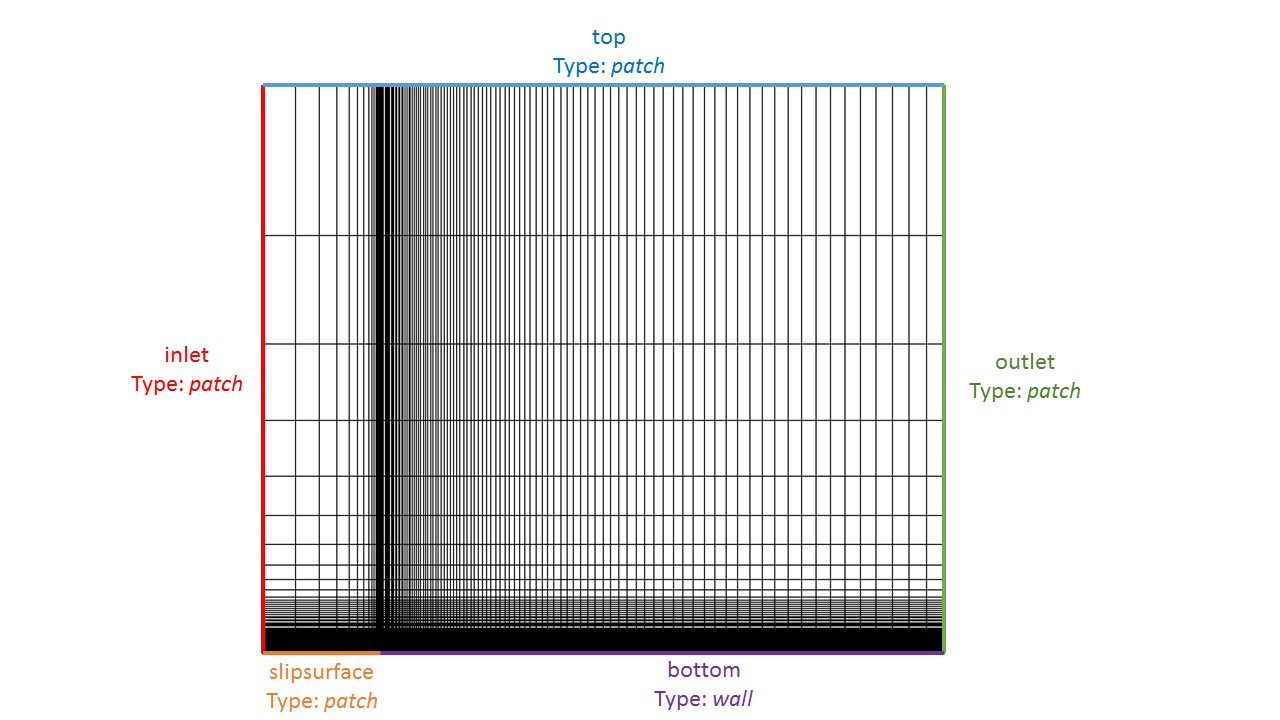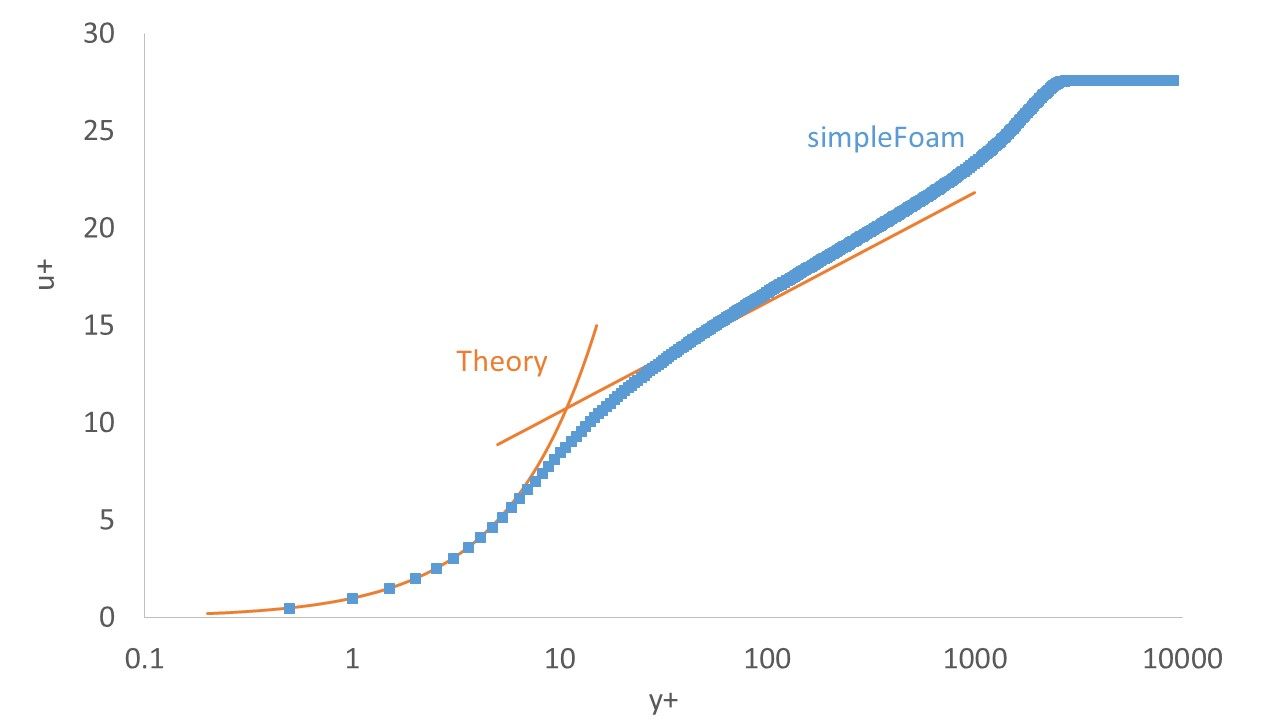Skip to content# Turbulent Zero Pressure Gradient Flat Plate – simpleFOAM, komegaSST

An excellent test case, and case to familiarize yourself with some of the turbulence models available in OpenFOAM is a 2D flat plate with zero pressure gradient. I will solve this problem using the solver simpleFoam and the komegaSST model.

Here are the sections of this post:

1. Quick Overview: kω-SST (komegaSST) Boundary Conditions
2. Case set-up and mesh
3. Results
• u+ vs. y+
• Coefficient of friction
4. Conclusions and useful resources

Download the case file here: kOmega Flat Plate Tutorial File

## Quick Overview: kω-SST (komegaSST) Boundary Conditions

In this section I will describe the boundary set-up for komegaSST  where no wall functions are implemented. This requires that the y+ along the wall is less than or equal to one. For the komegaSST turbulence model the boundary conditions are as follows:

At the wall:

• ω (omega) – specific dissipation rate
• BC type: fixedValue
• BC value:$\omega_{wall}=10\frac{6\nu_\infty}{\beta_1\left(\Delta y_{wall}\right)^2}$
• k – turbulent kinetic energy
• BC type: fixedValue
• BC value: 0
• nut – turbulent viscosity
• BC type: fixedValue
• BC value: 0

In the free-stream:

• ω (omega) – specific dissipation rate
• BC type: fixedValue
• BC value:$\frac{U_\infty}{L} < \omega_{\infty} < \frac{10 U_\infty}{L}$
• k – turbulent kinetic energy
• BC type: fixedValue
• BC value:$\frac{10^{-5}U_\infty^2}{Re_L} < k_{\infty} < \frac{0.1U_\infty^2}{Re_L}$
• nut – turbulent viscosity
• BC type: calculated
• BC value: 0 (this is just an initial value)

where$\beta_1=0.075$, and$\Delta y_{wall}$ is the normal distance from the wall to the first cell center.

## Case set-up and mesh

### Free-Stream Properties

For this case I have followed a similar set up to the 2D flat plate case used on the NASA turbulence modelling resource website. By doing this it gives me something to compare to! However, I am not going to use the exact set-up on that website. Since we are using simpleFoam, I am going to set up this case the way I prefer which is to use a velocity (U) of 1 m/s and scale all other properties accordingly. The simulation properties that I used are :

• U∞=1 m/s
• ν=4e-7 m2/s
• L=2 m

These correspond to a Reynolds number at L=2m of 5 million .

### Grid Generation

The grid used was generated in blockMesh. High inflation was used in the boundary layer region in order to achieve the desired y+ value of less than 1. For more details on grid generation using blockMesh see the OpenFOAM manual!Grid

### Boundary Conditions

For the incompressible solver simpleFoam, the minimum boundary conditions required for a simulation are p and U. However, if the simulation is a RANS simulation additional boundary conditions are required. For the kω-SST model we need to have a boundary condition on k and ω as well. The boundary conditions I defined in the zero (0) folder can be found in the attached tutorial file.

The only boundary condition that really needs any comment is omega. We calculate omega using$\omega_{wall}=10\frac{6\nu_\infty}{\beta_1\left(\Delta y_{wall}\right)^2}$. In our case the wall distance to the first cell center is 5.48316E-06. Using our free stream viscosity of 4E-7 this gives a value of omega at the wall of 10643595.39.

### Tip for fvSolution

If you find that the results you are getting are wrong, it could be that the residuals for the different properties are too high! Certain properties converge before others and therefore you need to ensure that they all converge to a sufficiently low value!

## Results

First we compare the coefficient of friction to the .dat file available from the .DAT available from the NASA Turbulence modelling resource. NOTE: We simulated at Reynolds number of 5 million whereas the NASA setup is at 10 million. So the x coordinate in the following is plots is rescaled and in fact we are only using half of the data from the .DAT file!Coefficient of Friction

We can see from the figure that the coefficient of friction from our simulation matches the expected data closely! Hurray!

Next let’s compare the u+ vs. y+ profile to the  universal profile for turbulent boundary layers. Recall that u+ is the velocity normalized by the friction velocity ($u_*$), and y+ is given by the following equation:$y^+ = \frac{u_* y}{\nu}$

The u+ is vs y+ is plotted here:u+ vs y+

We can see from the figure that our solution is pretty good! The y+ value of the first node is located around a y+ of approximately 0.5, the viscous sublayer matches very closely and the log law layer is not significantly off!

## Conclusions

In this post we simulated a zero pressure gradient flat plate at a Reynolds number of 5 million. We compared the results for shear stress to the NASA turbulence modelling resource expected results and showed close agreement. Then the u+ vs y+ profile was compared to the universal law of the wall and again the results were okay!

## Some useful references

The NASA Turbulence Modelling Resource, http://turbmodels.larc.nasa.gov/

Categories

Tags## 9 thoughts on “Turbulent Zero Pressure Gradient Flat Plate – simpleFOAM, komegaSST” Leave a comment ›

1.Eric Blair says:

To all asking about how to create the plot, the CFD solution will generate the streamwise velocity profile for you. All you have to do is plot it in terms of u+ and y+. You can use the same friction velocity calculated using the law of the wall for this purpose.

2.tareqkh says:

Hi,

What would be a typical y+ to produce u+ vs y+ for a flat plate if you used k-epsilon model instead? Would the results match the theory results?

Look forward to hearing from you,

3.varsey says:

Hi! it’s a realy great blog! But how can I subscribe to the new posts of yours? I dont want to miss something important, but cant find “subscribe” button.

•curiosityFluidsAdmin1 says:

Hi! Thanks for your interest in curiosityFluids! I have just added a follow button to the webpage! You can follow through wordpress, or by email 🙂

4.Tariq says:

Which surface are you referring to? Can you be more specific?

Best,

5.Tariq says:

Hello,

Thank you for the nice example. I have two questions about beta that you used to calculate omega at the wall. I saw that you used beta_1=0.075. Is this constant suitable for any case as long as we use y+<1?

The second question is regarding the y+ vs u+ plot. I have been trying to reproduce the figure but not luck yet. I used yPlusRAS and wallShearStress. Can you provide a step by step to get that plot?

Thank you for the great example.

•curiosityFluidsAdmin1 says:

The constant Beta_1 is a model constant. You can find the model constants at a reference website such as: https://turbmodels.larc.nasa.gov/sst.html

Or in the original paper:

Menter, F. R., “Two-Equation Eddy-Viscosity Turbulence Models for Engineering Applications,” AIAA Journal, Vol. 32, No. 8, August 1994, pp. 1598-1605

•curiosityFluidsAdmin1 says:

To get the u+ vs. y+ you must extract a line normal to the surface. Then use the information along the line to calculate u+ and y+ and plot them against each other. See more on the law of the wall here: https://en.wikipedia.org/wiki/Law_of_the_wall

•varsey says:

I have the exact same question. Did you managed to create this plot?

This site uses Akismet to reduce spam. Learn how your comment data is processed.